# Select the first set of parametric equations, x = a cos(bt), y = c sin(dt). (a)...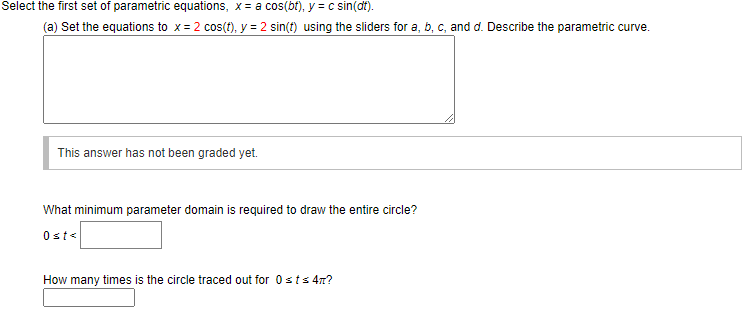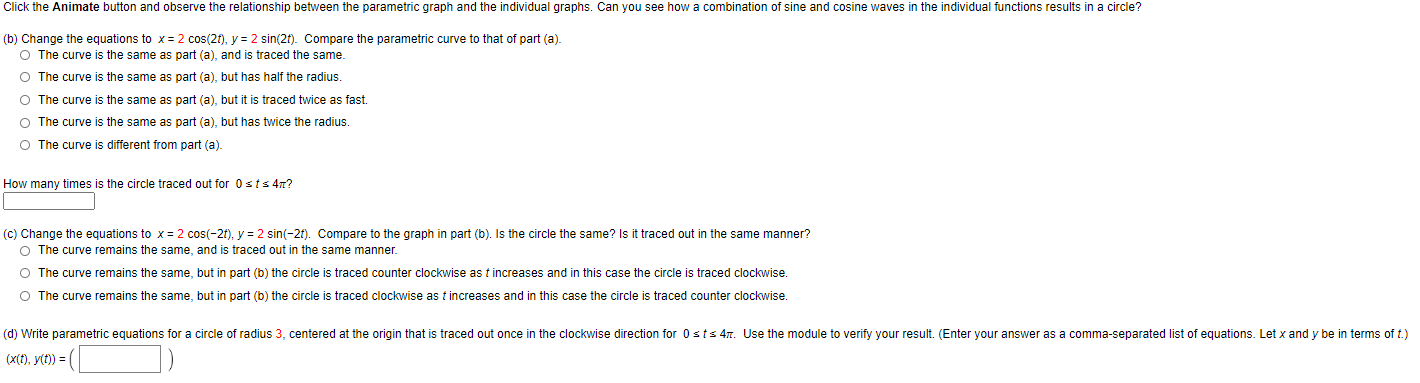Select the first set of parametric equations, x = a cos(bt), y = c sin(dt). (a) Set the equations to x = 2 cos(t), y = 2 sin(t) using the sliders for a, b, c, and d. Describe the parametric curve. This answer has not been graded yet. What minimum parameter domain is required to draw the entire circle? Osts How many times is the circle traced out for Osts 4?
Click the Animate button and observe the relationship between the parametric graph and the individual graphs. Can you see how a combination of sine and cosine waves in the individual functions results in a circle? (b) Change the equations to x = 2 cos(21), y = 2 sin(2t). Compare the parametric curve to that of part (a). O The curve is the same as part (a), and is traced the same. O The curve is the same as part (a), but has half the radius. O The curve is the same as part (a), but it is traced twice as fast. The curve is the same as part (a), but has twice the radius. O The curve is different from part (a). How many times is the circle traced out for Osts 47? (C) Change the equations to x = 2 cos(-21), y = 2 sin(-26). Compare to the graph in part (b). Is the circle the same? Is it traced out in the same manner? O The curve remains the same, and is traced out in the same manner. O The curve remains the same, but in part (b) the circle is traced counter clockwise as t increases and in this case the circle is traced clockwise. O The curve remains the same, but in part (b) the circle is traced clockwise as t increases and in this case the circle is traced counter clockwise. (d) Write parametric equations for a circle of radius 3, centered at the origin that is traced out once in the clockwise direction for Osts 47. Use the module to verify your result. (Enter your answer as a comma-separated list of equations. Let x and y be in terms of t.) (X(t), y(t)) =(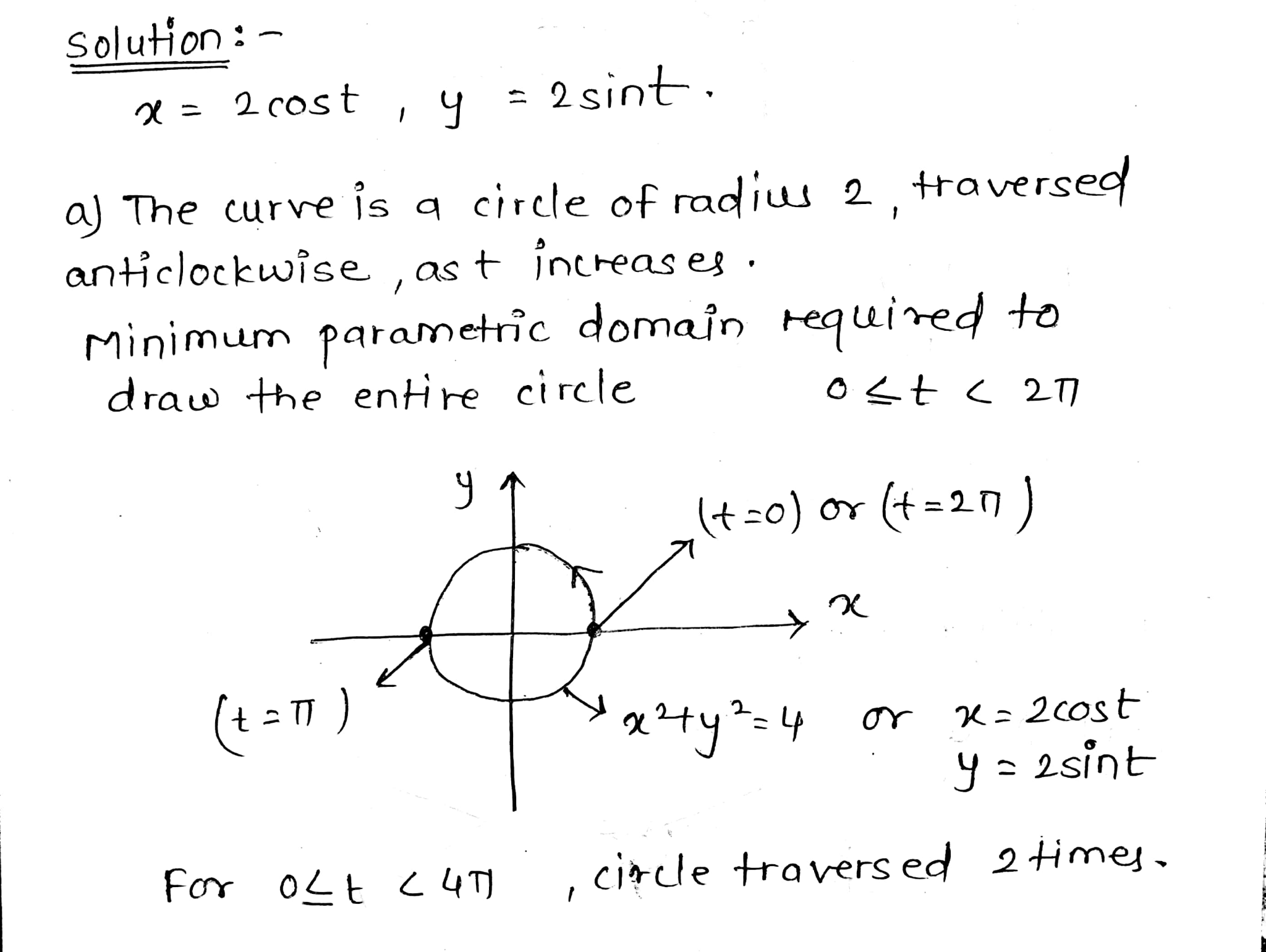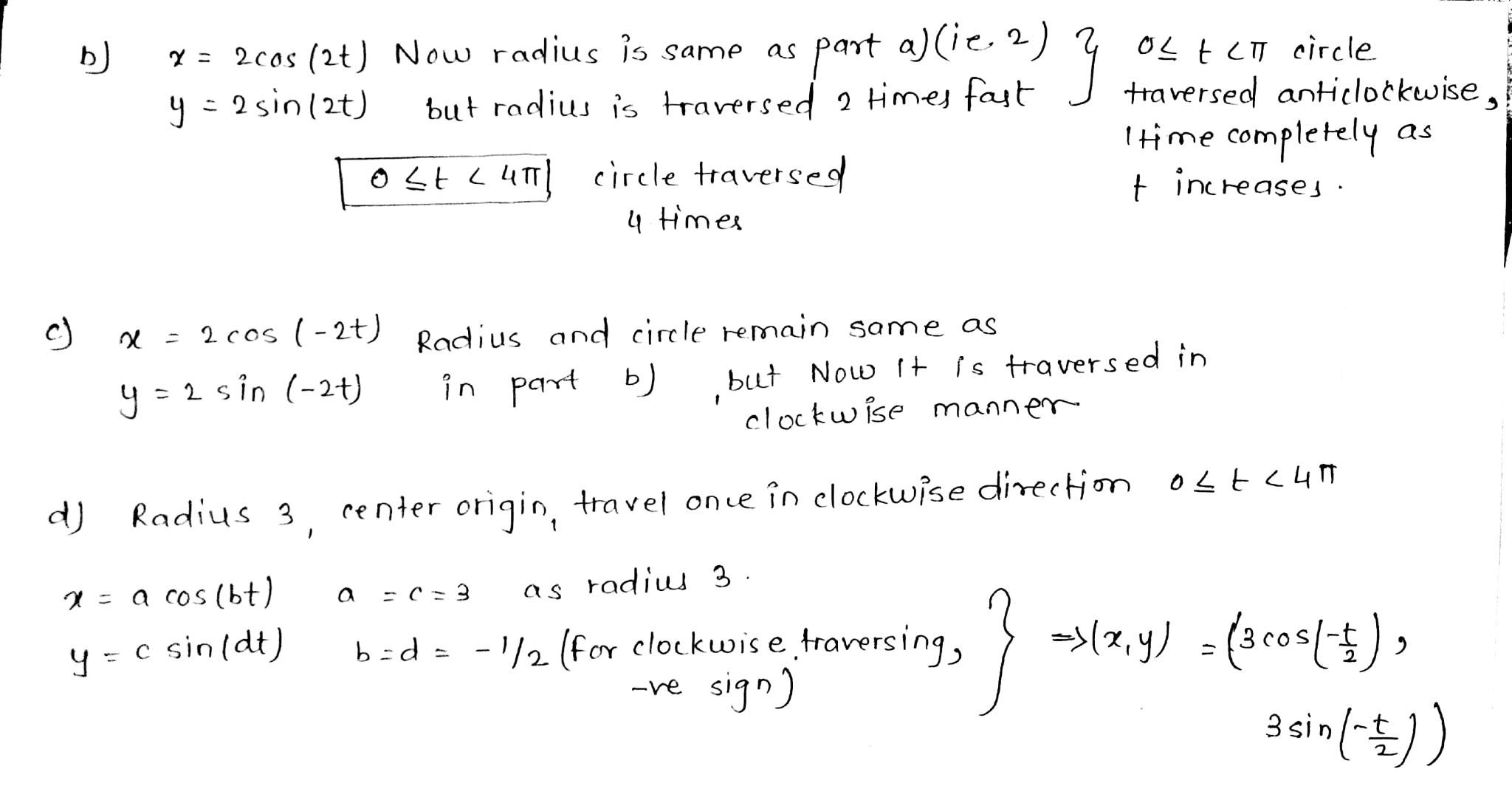#### Earn Coin

Coins can be redeemed for fabulous gifts.

Similar Homework Help Questions
• ### Find parametric equations (not unique) for the following circle and give an interval for the parameter....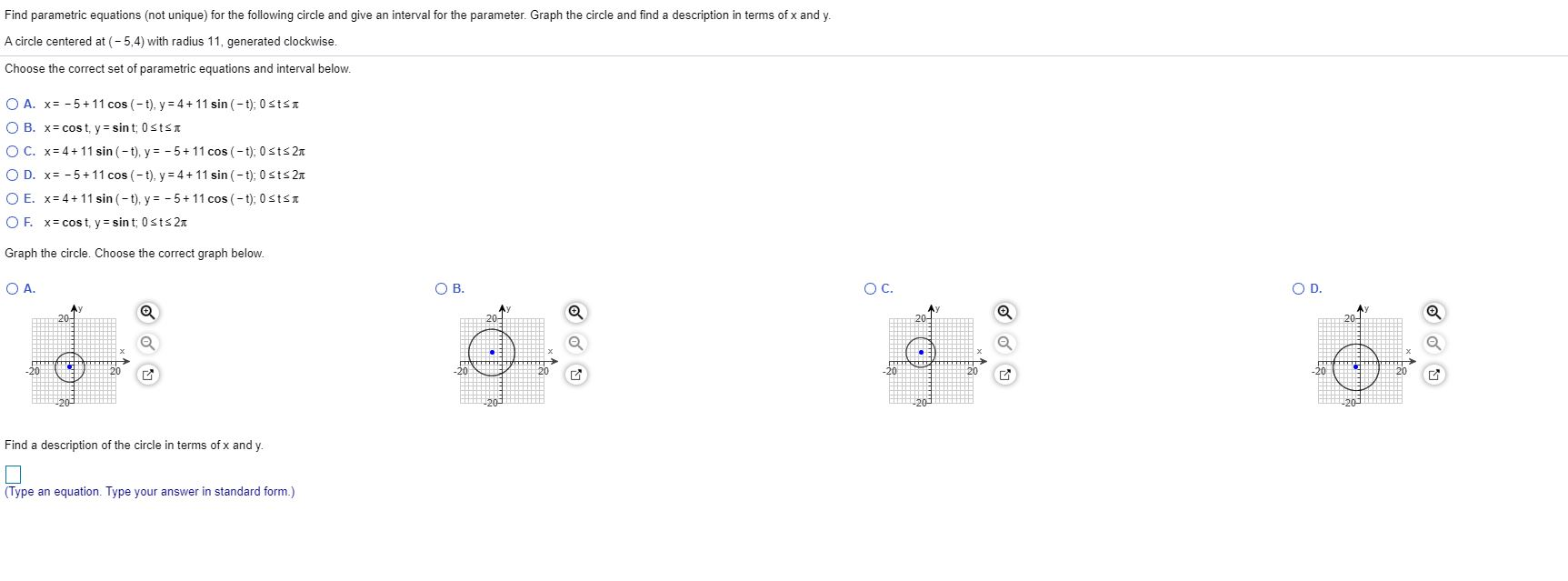Find parametric equations (not unique) for the following circle and give an interval for the parameter. Graph the circle and find a description in terms of x and y. A circle centered at (-5,4) with radius 11, generated clockwise. Choose the correct set of parametric equations and interval below. O A. x= -5+11 cos(-t), y = 4 + 11 sin(-t): 0 SISI OB. x= cost, y = sint: Ostst OC. x= 4 + 11 sin(-t), y = -5 + 11...

• ### Give parametric equations that describe a full circle of radius​ R, centered at the origin with...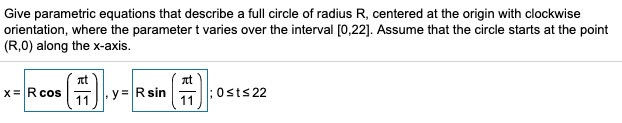Give parametric equations that describe a full circle of radius​ R, centered at the origin with clockwise​ orientation, where the parameter t varies over the interval ​[0,22​]. Assume that the circle starts at the point​ (R,0) along the​ x-axis. Consider the following parametric​ equations, x=−t+7​, y=−3t−3​; minus−5less than or equals≤tless than or equals≤5. Complete parts​ (a) through​ (d) below. Consider the following parametric equation. a.Eliminate the parameter to obtain an equation in x and y. b.Describe the curve and indicate...

• ### Find parametric equations for the path of a particle that moves around the given circle in...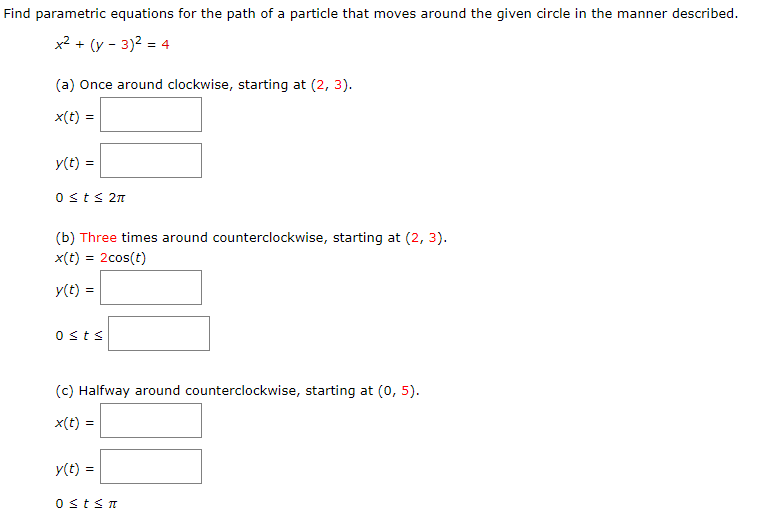Find parametric equations for the path of a particle that moves around the given circle in the manner described. x2 + (y - 3)2 = 4 (a) Once around clockwise, starting at (2, 3). X(t) = y(t) = Osts 211 (b) Three times around counterclockwise, starting at (2, 3). X(t) = 2cos(t) y(t) = Osts (c) Halfway around counterclockwise, starting at (0,5). x(t) = y(t) = Osts

• ### 7. [-16 Points) DETAILS SCALCCC4 1.7.031. Find parametric equations for the path of a particle that...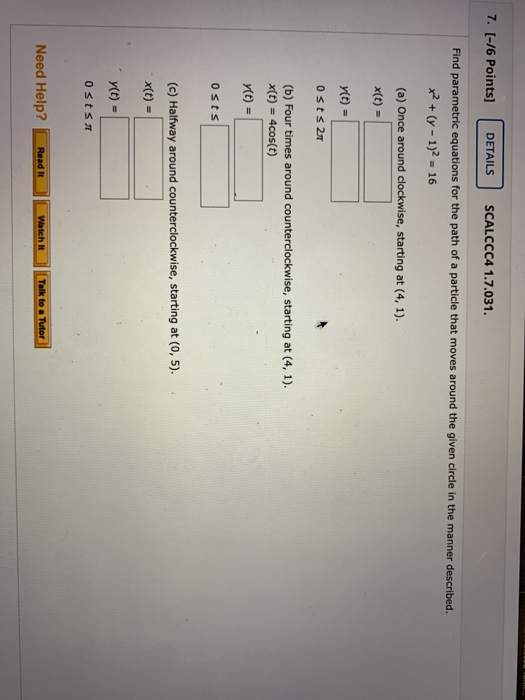7. [-16 Points) DETAILS SCALCCC4 1.7.031. Find parametric equations for the path of a particle that moves around the given circle in the manner described. x2 + (y - 1)2 = 16 (a) Once around clockwise, starting at (4,1). X(t) = (t) = Osts 2017 (b) Four times around counterclockwise, starting at (4,1). x(t) = 4cos(t) (t) = osts (c) Halfway around counterclockwise, starting at (0,5). x(t) = y(t) = osts Need Help? Read it Watch Talk to Tutor

• ### Evaluate Sc (2+2)dy where C is described by parametric equations x(t) = cos(t), y= sin(t), z...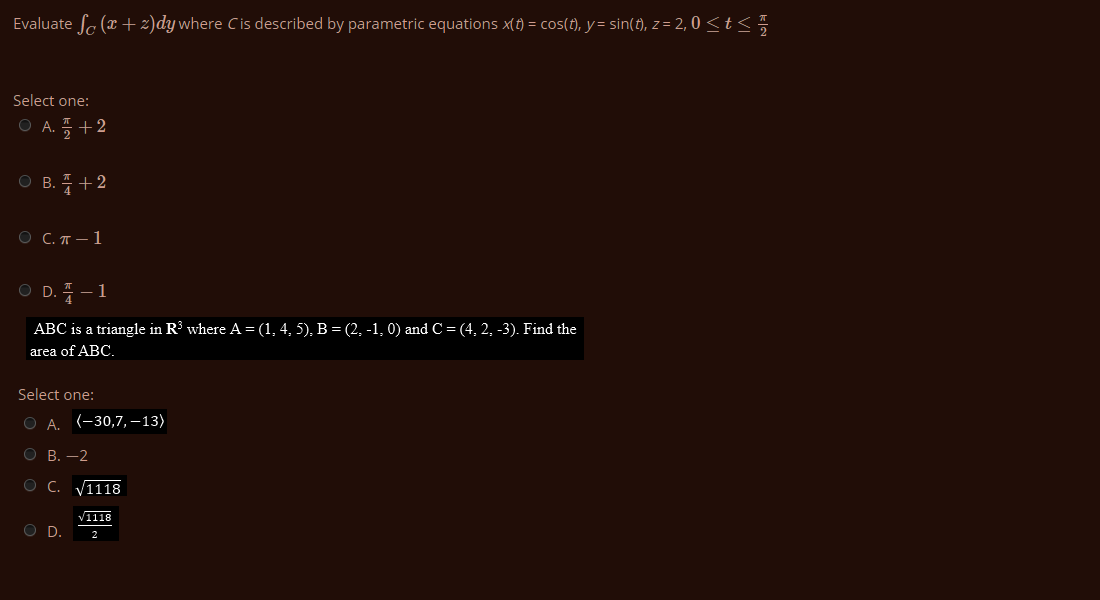Evaluate Sc (2+2)dy where C is described by parametric equations x(t) = cos(t), y= sin(t), z = 2,0 <t< Select one: O A. +2 O B. 1+2 O C.-1 OD. -1 ABC is a triangle in R where A =(1,4,5), B =(2,-1,0) and C =(4, 2, -3). Find the area of ABC. Select one: O A. (-30,7, -13) O B. -2 OC. V1118 O D. VILLE

• ### An object is moving around the unit circle with parametric equations x(t)=cos(t), y(t)=sin(t), so it's location...

An object is moving around the unit circle with parametric equations x(t)=cos(t), y(t)=sin(t), so it's location at time t is P(t)=(cos(t),sin(t)) . Assume 0 < t < ?/2. At a given time t, the tangent line to the unit circle at the position P(t) will determine a right triangle in the first quadrant. (Connect the origin with the y-intercept and x-intercept of the tangent line.)

• ### 4. Eliminate the parameter for the given set of parametric equations then sketch the graph of...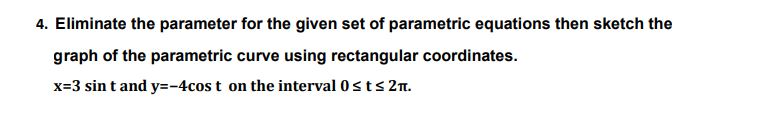4. Eliminate the parameter for the given set of parametric equations then sketch the graph of the parametric curve using rectangular coordinates. x=3 sin t and y=-4cost on the interval Osts 2tt.

• ### (a) Give a set of parametric equations (with domain) for the line segment from (4, -1)...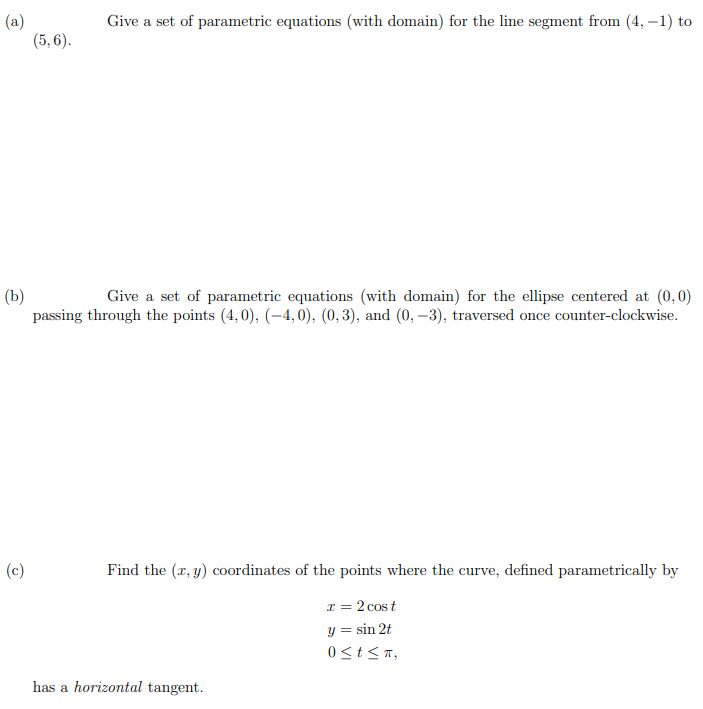(a) Give a set of parametric equations (with domain) for the line segment from (4, -1) to (5,6). (b) Give a set of parametric equations (with domain) for the ellipse centered at (0,0) passing through the points (4,0), (-4,0), (0,3), and (0, -3), traversed once counter-clockwise. (c) Find the (x, y) coordinates of the points where the curve, defined parametrically by I= 2 cost y = sin 2t 0<t<T, has a horizontal tangent.

• ### Consider the parametric equations below x-vi y-2.1 (a) Sketch the curve by using the parametric equations...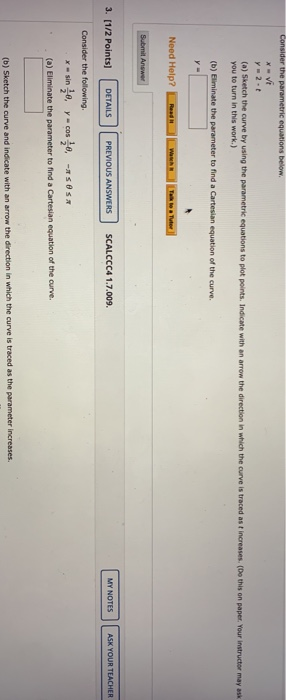Consider the parametric equations below x-vi y-2.1 (a) Sketch the curve by using the parametric equations to plot points. Indicate with an arrow the direction in which the curve is traced as t increases. (Do this on paper. Your instructor may ask you to turn in this work.) (b) Eliminate the parameter to find a Cartesian equation of the curve. y- Need Help? Submit Answer 3. [1/2 points) DETAILS PREVIOUS ANSWERS SCALCCC4 1.7.009. MY NOTES ASK YOUR TEACHER Consider the...

• ### For parts e)-g), consider parametric equations x=6 sint and y=-6cost. They produce a circle centered at...For parts e)-g), consider parametric equations x=6 sint and y=-6cost. They produce a circle centered at the origin. At time t = 0 seconds, a particle starts moving along this circle. True or False? e) True The radius of the circle is 6. f) The start point is on the negative side of the y-axis. The particle moves counter-clockwise.